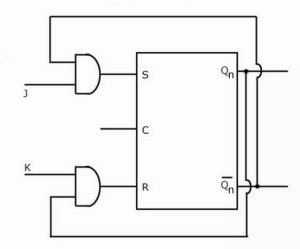# Conversion of SR Flip Flop to JK and D Flip Flop

Hello friends, in this article, we will learn Conversion of SR flip flop to JK and D flip flop. We will cover, introduction, Conversion tables, Logic diagrams, and K-maps for the same.

## Conversion of SR Flip Flop

### Introduction:

For the conversion of one type of flip flop into another type of flip flop, we are required to design a combinational circuit. The inputs of the required flip flop are given to this combinational circuit. The output of combinational circuit is nothing but the input of the actual flip flop which we are going to convert into another type. Then the output of the actual flip is the output of required flip flop.

### Conversion of SR flip flop:

We can convert SR flip flop into JK and T type of flip flop.

### 1)      SR flip flop to JK flip flop:

The following figure shows the conversion table, K-maps, and Logic diagram for the conversion of SR flip flop to JK flip flop.

K-Map

[caption id="" align="alignnone" width="300"]Logic diagram[/caption]

In this case we are required to convert SR flip flop into JK flip flop. Therefore we have to first design and connect the combinational circuit to the input of SR flip flop so that it will produce same outputs as that of JK flip flop. Here the external inputs are J and K. S and R will be the outputs of designed combinational circuit which are inputs of actual flip flop. We write a truth table with J, K, Qn, Qn+1, S and R. where Qn is the present state of the flip flop and Qn+1 will be the next state obtained when the particular J and K inputs are applied.

J, K and Qn can have eight combinations. For each combination of J, K and Qn find the corresponding Qn+1, i.e. determine to which next state the JK flip flop will go from the present state Qn if the present inputs J and K are applied. Now complete the table by writing the values of S and R required to get each Qn+1 from the corresponding Qn. i.e. write what values of S and R are required to change the state of the flip flop from Qn to Qn+1.

### 2)      SR flip flop to D flip flop:

Similarly, for the conversion of SR flip flop into the D flip flop, we have connected the combinational circuit to the inputs of the SR flip flop. In this case D the external input of the circuit. The output of the combinational circuit is connected to the inputs of the actual flip flop i.e. SR flip flop. Then the output of this flip flop will be the same as D flip flop.

The conversion table, K-maps, and Logic diagram for the conversion of SR flip flop to D flip flop.Logic Diagram